# Selina Concise Solutions for Chapter 17 Special Types of Quadrilaterals Class 8 ICSE Mathematics

##1. In parallelogram ABCD, ∠A = 3 times ∠B. Find all the angles of the parallelogram. In the same parallelogram, if AB = 5x – 7 and CD = 3x + 1 ; find the length of CD.
Solution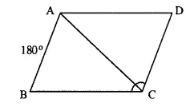Let ∠B = x
∠A = 3 ∠B = 3x
∠A + ∠B = 180°
3x + x = 180°
⇒ 4x = 180°
⇒ x = 45°
∠B = 45°
∠A = 3x = 3 x 45 = 135°
and ∠B = ∠D = 45°
opposite angles of ||gm are equal.
∠A = ∠C = 135°
opposite sides of //gm are equal.
AB = CD
5x – 7 = 3x + 1
⇒ 5x – 3x = 1+7
⇒ 2x = 8
⇒ x = 4°
CD = 3 x 4 + 1 = 13
Hence, 135°, 45°, 135°  and  45°; 13

2. In parallelogram PQRS, ∠Q = (4x – 5)° and ∠S = (3x + 10)°. Calculate : ∠Q and ∠R.
Solution
In parallelogram PQRS,
∠Q = (4x – 5)° and ∠S = (3x + 10)°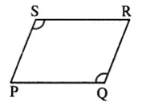opposite ∠s of //gm are equal.
∠Q = ∠S
4x – 5 = 3x + 10
⇒ 4x – 3x = 10 + 5
⇒ x = 15
∠Q = 4x – 5 = 4×15 – 5 = 55°
Also ∠Q + ∠R = 180°
⇒ 55° + ∠R = 180°
∠R = 180° - 55° = 125°
∠Q = 55° ; ∠R = 125°

3. In rhombus ABCD ;
(i) if ∠A = 74° ; find ∠B and ∠C.
(ii) if AD = 7.5 cm ; find BC and CD.
Solution
∠A + ∠B = 180°
⇒ 74° + ∠B = 180°
⇒ ∠B =  180° – 74° = 106°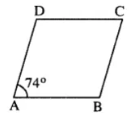opposite angles of Rhombus are equal.
∠A = ∠C = 74°
Sides of Rhombus are equal.
BC = CD = AD = 7.5 cm
(i) ∠B = 106° ; ∠C = 74°
(ii) BC = 7.5 cm and CD = 7.5 cm

4. In square PQRS :
(i) if PQ = 3x – 7 and QR = x + 3 ; find PS
(ii) if PR = 5x and QR = 9x – 8. Find QS
Solution
(i) sides of square are equal.PQ = QR
⇒ 3x – 7 = x + 3
⇒ 3x – x = 3 + 7
⇒ 2x = 10
⇒ x = 5
PS = PQ = 3x – 7 = 3×5 – 7 =8

(ii) PR = 5x and QS = 9x – 8As diagonals of square are equal.
PR = QS
5x = 9x – 8
⇒ 5x – 9x = -8
⇒ -4x = -8
⇒ x = 2
QS = 9x – 8 = 9 ×2 – 8 = 10

5. ABCD is a rectangle, if ∠BPC = 124°Solution
Diagonals of rectangle are equal and bisect each other.
∠PBC = ∠PCB = x (say)
But ∠BPC + ∠PBC + ∠PCB = 180°
124° + x + x = 180°
⇒ 2x = 180° – 124°
⇒ 2x = 56°
⇒ x = 28°
∠PBC = 28°
But ∠PBC = ∠ADP [Alternate ∠s]
Again ∠APB = 180° – 124° = 56°
Also PA = PB
∠BAP =  1/2 (180° – ∠APB)
= 1/2 ×(180°- 56°)
=  1/2 ×124° = 62°
Hence (i) ∠BAP = 62° (ii) ∠ADP = 28°

6. ABCD is a rhombus. If ∠BAC = 38°, find :
(i) ∠ACB
(ii) ∠DAC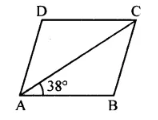Solution
ABCD is Rhombus (Given)
AB = BC
∠BAC = ∠ACB (∠s opp. to equal sides)
But ∠BAC = 38° (Given)
∠ACB = 38°
In ∆ABC,
∠ABC + ∠BAC + ∠ACB = 180°
⇒ ∠ABC + 38° + 38° = 180°
⇒ ∠ABC = 180° – 76° = 104°
But ∠ABC = ∠ADC (opp. ∠s of rhombus)
⇒ ∠DAC = ∠DCA ( AD = CD)
⇒ ∠DAC =  1/2 [180° – 104°]
⇒ ∠DAC =  1/2 x 76° = 38°
Hence (i) ∠ACB = 38° (ii) ∠DAC = 38° (iii) ∠ADC = 104°

7. ABCD is a rhombus. If ∠BCA = 35°. find ∠ADC.
Solution
Given: Rhombus ABCD in which ∠BCA = 35°∠DAC = ∠BCA (Alternate ∠s)
But ∠BCA = 35° (Given)
∠DAC = 35°
But ∠DAC = ∠ACD  (AD = CD)
& ∠DAC +∠ACD + ∠ADC = 180°
⇒ 35° + 35° + ∠ADC = 180°
⇒ ∠ADC = 180° – 70° = 110°

8. PQRS is a parallelogram whose diagonals intersect at M.
If ∠PMS = 54°, ∠QSR = 25° and ∠SQR = 30° ; find :
(i) ∠RPS
(ii) ∠PRS
(iii) ∠PSR.
Solution
Given : ||gm PQRS in which diagonals PR & QS intersect at M.
∠PMS = 54° ; ∠QSR = 25° and ∠SQR = 30°To find : (i) ∠RPS (ii) ∠PRS (iii) ∠PSR
Proof : QR || PS
∠PSQ = ∠SQR (Alternate ∠s)
But ∠SQR = 30° (Given)
∠PSQ = 30°
In ∆SMP,
∠PMS + ∠PSM + ∠MPS = 180° or 54° + 30° + ∠RPS = 180°
⇒ ∠RPS = 180°- 84° = 96°
Now ∠PRS + ∠RSQ = ∠PMS
∠PRS + 25° = 54°
⇒ ∠PRS = 54° – 25° = 29°
⇒ ∠PSR = ∠PSQ + ∠RSQ = 30° + 25° = 55°
Hence (i) ∠RPS = 96° (ii) ∠PRS = 29° (iii) ∠PSR = 55°

9. Given: Parallelogram ABCD in which diagonals AC and BD intersect at M.
Prove: M is mid-point of LN.
SolutionProof: Diagonals of //gm bisect each other.
MD = MB
Also ∠ADB = ∠DBN (Alternate ∠s)
& ∠DML = ∠BMN (Vert. opp. ∠s)
∆DML = ∆BMN
LM = MN
M is mid-point of LN.
Hence proved.

10. In an Isosceles-trapezium, show that the opposite angles are supplementary.
SolutionGiven: ABCD is isosceles trapezium in which AD = BC
To Prove : (i) ∠A + ∠C = 180°
(ii) ∠B + ∠D = 180°
Proof: AB || CD
⇒ ∠A + ∠D = 180°
But ∠A = ∠B  [Trapezium is isosceles]
∠B + ∠D = 180°
Similarly ∠A + ∠C = 180°
Hence the result.

11. ABCD is a parallelogram. What kind of quadrilateral is it if :
(i) AC = BD and AC is perpendicular to BD?
(ii) AC is perpendicular to BD but is not equal to it?
(iii) AC = BD but AC is not perpendicular to BD?
Solution
(i)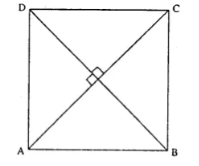AC = BD (Given)
& AC ⊥ BD  (Given)
i.e., Diagonals of quadrilateral are equal and they are ⊥r to each other.
∴ ABCD is square.

(ii)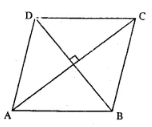AC ⊥BD (Given)
But  AC & BD are not equal
∴ ABCD is a Rhombus.

(iii)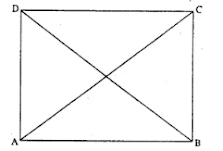AC = BD  but Ac & BD are not ⊥r to each other.
∴ ABCD  is a rectangle.

12. Prove that the diagonals of a parallelogram bisect each other.
SolutionGiven: ||gm ABCD in which diagonals AC and BD bisect each other.
To Prove: OA = OC and OB = OD
Proof: AB || CD (Given)
∠1 = ∠2 (alternate ∠s)
∠3 = ∠4 (alternate ∠s)
and AB = CD (opposite sides of //gm)
∆COD = ∆AOB (A.S.A. rule)
OA = OC and OB = OD
Hence the result.

13. If the diagonals of a parallelogram are of equal lengths, the parallelogram is a rectangle. Prove it.
SolutionGiven: //gm ABCD in which AC = BD
To Prove : ABCD is rectangle.
Proof :
In ∆ABC and ∆ABD
AB = AB (Common)
AC = BD (Given)
BC = AD (opposite sides of ||gm)
∆ABC = ∆ABD (S.S.S. Rule)
∠A = ∠B
But AD // BC (opp. sides of ||gm are ||)
∠A + ∠B = 180°
∠A = ∠B = 90°
Similarly, ∠D = ∠C = 90°
Hence, ABCD is a rectangle.

14. In parallelogram ABCD, E is the mid-point of AD and F is the mid-point of BC. Prove that BFDE is a parallelogram.
SolutionGiven: //gm ABCD in which E and F are mid-points of AD and BC respectively.
To Prove: BFDE is a ||gm.
Proof :
E is mid-point of AD. (Given)
Also F is mid-point of BC (Given)
BF =  1/2 BC
But AD = BC (opp. sides of ||gm)
BF = DE
⇒ DE || BF
Now DE || BF and DE = BF
Hence BFDE is a ||gm.

15. In parallelogram ABCD, E is the mid-point of side AB and CE bisects angle BCD. Prove that :
(ii) DE bisects and ∠ADC and
(iii) Angle DEC is a right angle.
Solution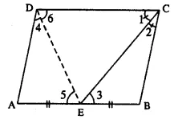Given: ||gm ABCD in which E is mid-point of AB and CE bisects ZBCD.
To Prove : (i) AE = AD
(iii) ∠DEC = 90°
Const. Join DE
Proof :
(i) AB || CD (Given)
and CE bisects it.
∠1 = ∠3 (alternate ∠s) …(i)
But ∠1 = ∠2 (Given) ...(ii)
From (i) & (ii)
∠2 = ∠3
BC = BE (sides opp. to equal angles)
But BC = AD (opp. sides of ||gm)
and BE = AE (Given)
∠4 = ∠5 (∠s opp. to equal sides)
But ∠5 = ∠6 (alternate ∠s)
⇒ ∠4 = ∠6
⇒ ∠D + ∠C = 180°
⇒ 2∠6 + 2∠1 = 180°
DE and CE are bisectors.
∠6 + ∠1 = 180°/2
⇒ ∠6 + ∠1 = 90°
But ∠DEC + ∠6 + ∠1 = 180°
⇒ ∠DEC + 90° = 180°
⇒ ∠DEC = 180° – 90°
⇒ ∠DEC = 90°
Hence the result.

16. In the following diagram, the bisectors of interior angles of the parallelogram PQRS enclose a quadrilateral ABCD.Show that:
(i) ∠PSB + ∠SPB = 90°
(ii) ∠PBS = 90°
(iii) ∠ABC = 90°
(v) ∠A = 90°
(vi) ABCD is a rectangle
Thus, the bisectors of the angles of a parallelogram enclose a rectangle.
Solution
Given: In parallelogram ABCD bisector of angles P and Q, meet at A, bisectors of ∠R and ∠S meet at C. Forming a quadrilateral ABCD as shown in the figure.
To prove:
(i) ∠PSB + ∠SPB = 90°
(ii) ∠PBS = 90°
(iii) ∠ABC = 90°
(v) ∠A = 9°
(vi) ABCD is a rectangle
Proof:
In parallelogram PQRS,
PS || QR (opposite sides)
∠P + ∠Q = 180°
and AP and AQ are the bisectors of consecutive angles ∠P and ∠Q of the parallelogram
∠APQ + ∠AQP =  1/2 ×180° = 90°
But in ∆APQ,
∠A + ∠APQ + ∠AQP = 180° (Angles of a triangle)
⇒ ∠A + 90° = 180°
⇒ ∠A = 180° – 90°
(v) ∠A = 90°
Similarly PQ || SR
∠PSB + SPB = 90°
(ii) and ∠PBS = 90°
But, ∠ABC = ∠PBS (Vertically opposite angles)
(iii) ∠ABC = 90°
Similarly we can prove that
(iv) ∠ADC = 90° and ∠C = 90°
(vi) ABCD is a rectangle (Each angle of a quadrilateral is 90°)
Hence proved.

17. In parallelogram ABCD, X and Y are midpoints of opposite sides AB and DC respectively. Prove that:
(i) AX = YC
(ii) AX is parallel to YC
(iii) AXCY is a parallelogram.
Solution
Given: In parallelogram ABCD, X and Y are the mid-points of sides AB and DC respectively AY and CX are joinedTo prove:
(i) AX = YC
(ii) AX is parallel to YC
(iii) AXCY is a parallelogram
Proof:
AB || DC and X and Y are the mid-points of the sides AB and DC respectively
(i) AX = YC (1/2 of opposite sides of a parallelogram)
(ii) and AX || YC
(iii) AXCY is a parallelogram (A pair of opposite sides are equal and parallel)
Hence proved.

18. The given figure shows parallelogram ABCD. Points M and N lie in diagonal BD such that DM = BN.Prove that:
(i) ∆DMC = ∆BNA and so CM = AN
(ii) ∆AMD = ∆CNB and so AM CN
(iii) ANCM is a parallelogram.
Solution
Given: In parallelogram ABCD, points M and N lie on the diagonal BD such that DM = BN
AN, NC, CM and MA are joined
To prove:
(i) ∆DMC = ∆BNA and so CM = AN
(ii) ∆AMD = ∆CNB and so AM = CN
(iii) ANCM is a parallelogram
Proof :
(i) In ∆DMC and ∆BNA.
CD = AB (opposite sides of ||gm ABCD)
DM = BN (given)
∠CDM = ∠ABN (alternate angles)
∆DMC = ∆BNA (SAS axiom)
CM = AN (c.p.c.t.)
Similarly, in ∆AMD and ∆CNB
AD = BC (opposite sides of ||gm)
DM = BN (given)
∆AMD = ∆CNB (SAS axiom)
AM = CN (c.p.c.t.)
(iii) CM = AN and AM = CN (proved)
ANCM is a parallelogram (opposite sides are equal)
Hence proved.

19. The given figure shows a rhombus ABCD in which angle BCD = 80°. Find angles x and y.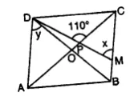Solution
In rhombus ABCD, diagonals AC and BD bisect each other at 90°
∠BCD = 80°
Diagonals bisect the opposite angles also ∠BCD = ∠BAD (Opposite angles of rhombus)
∠BAD = 80° and ∠ABC = ∠ADC = 180° – 80° = 100°
Diagonals bisect opposite angles
∠OCB or ∠PCB = 80°/2  = 40°
In ∆PCM,
Ext. CPD = ∠OCB + ∠PMC
⇒ 110° = 40° + x
⇒ x = 110° – 40° = 70°
Hence, x = 70° and y = 50°

20. Use the information given in the alongside diagram to find the value of x, y and z.Solution
ABCD is a parallelogram and AC is its diagonal which bisects the opposite angle
Opposite sides of a parallelogram are equal
3x + 14 = 2x + 25
⇒ 3x – 2x = 25 – 14
⇒ x = 11
∴ x = 11 cm
∠DCA = ∠CAB (Alternate angles)
y + 9° = 24
⇒ y = 24° – 9° = 15°
∠DAB = 3y° + 5° + 24° = 3×15 + 5 + 24° = 50° + 24°  = 74°
∠ABC = 180° - ∠DAB = 180° – 74° = 106°
z = 106°
Hence, x = 11 cm, y = 15°, z = 106°

21. The following figure is a rectangle in which x: y = 3 : 7; find the values of x and y.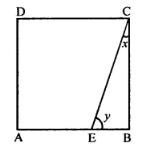Solution
ABCD is a rectangle,
x : y = 3 : 1
In ∆BCE, ∠B = 90°
x + y = 90°
But x : y = 3 : 7
Sum of ratios = 3 + 7 = 10
∴ x = (90˚× 3)/10 = 27˚
And y = (90˚ × 7)/10 = 63˚
Hence, x = 27˚, y = 63˚

22. In the given figure, AB // EC, AB = AC and AE bisects ∠DAC. Prove that: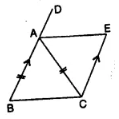(i) ∠EAC = ∠ACB
(ii) ABCE is a parallelogram.
Solution
ABCE is a quadrilateral in which AC is its diagonal and AB || EC, AB = AC
BA is produced to D
AE bisects ∠DAC
To prove:
(i) ∠EAC = ∠ACB
(ii) ABCE is a parallelogram
Proof:
(i) In ∆ABC and ∆ZAEC
AC=AC (common)
AB = CE (given)
∠BAC = ∠ACE (Alternate angle)
∆ABC = ∆AEC (SAS Axiom)

(ii) ∠BCA = ∠CAE (c.p.c.t.)
But these are alternate angles
AE || BC
But AB || EC (given)
∴ ABCD is a parallelogram